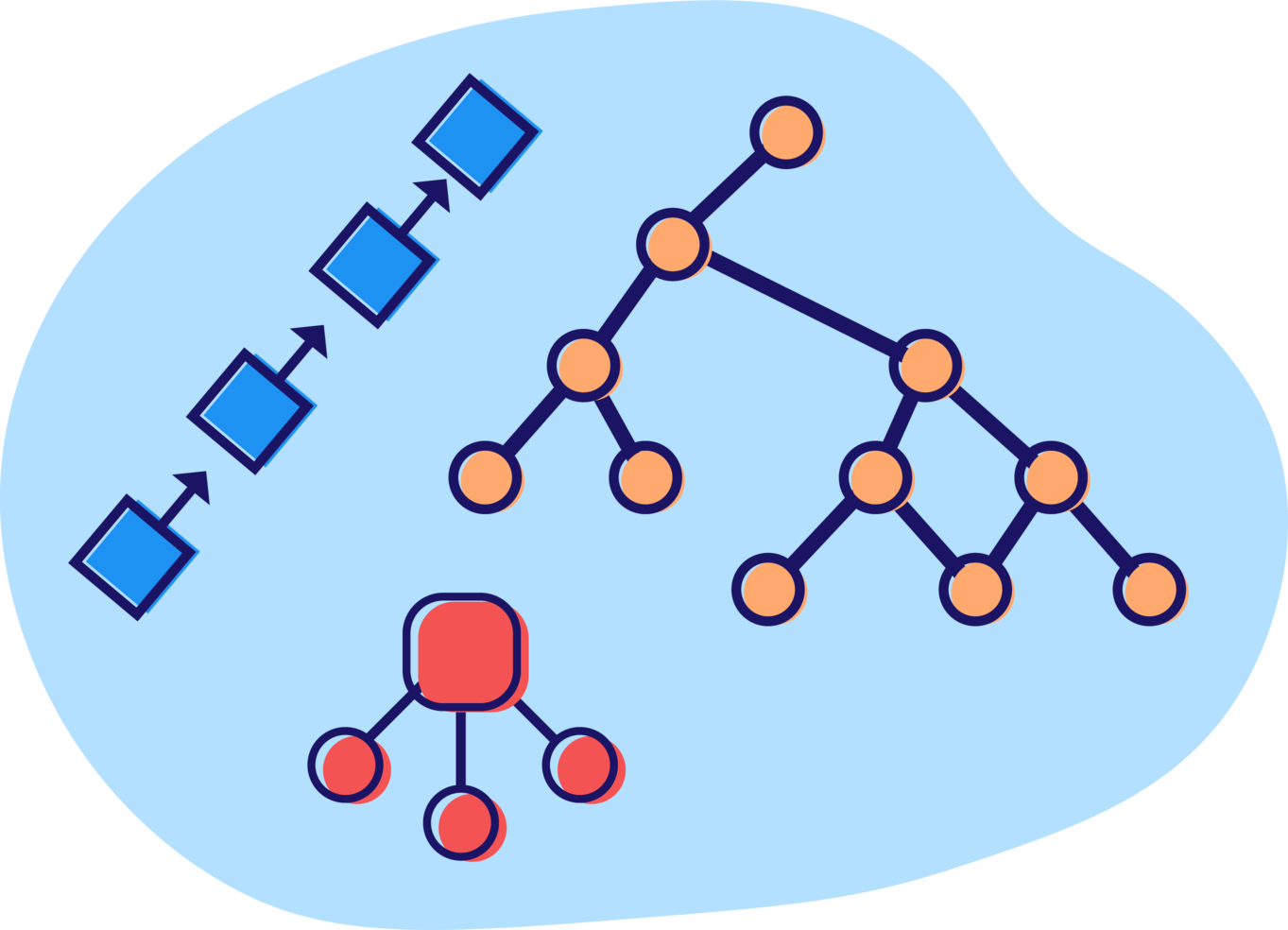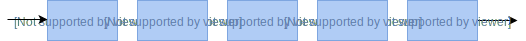Home/Blog/Programming/Top data structures and algorithms every developer must know

# Top data structures and algorithms every developer must know

Apr 23, 2020 - 16 min read
Aaron XieMany shudder at the thought of a coding interview. It can be stressful, grueling, and challenging. Oftentimes, it can be a struggle simply to know what topics to prepare.

Today, you will be introduced to the primary data structures and algorithms that are tested in a coding interview. After reading this article, you should have a good idea of what you need to prepare for to land your dream job.

We will cover the following:

## Why should you learn data structures and algorithms?

The coding interview tests for your problem-solving abilities and understanding of computer science concepts. Usually, you are given about 30 - 45 minutes to solve one complex problem.

This is where data structures and algorithms come in. These interviews will test you on topics such as linked lists, queues, sorting, searching, and much more, so it’s crucial to prepare.

Not only do companies want to test your technical knowledge, but they also want to evaluate your problem-solving skills.

If you get the job, you will often face bugs that you need to fix, and companies want to make sure that you can overcome these obstacles.

Furthermore, you will often use specific data structures and algorithms to optimize your code so that it runs as efficiently as possible.

## Understanding Big O notation

Big O notation is an asymptotic analysis that describes how long an algorithm takes to perform. In other words, it’s used to talk about how efficient or complex an algorithm is.

Big O describes the execution time, or run time, of an algorithm relative to its input $N$ as the input increases. Though we can analyze an algorithm’s efficiency using average-case and best-case, we typically use worst-case with Big O notation.

Today, you will learn about time complexity, but take note that space complexity is also an important concept to understand. Runtime complexity can be a difficult concept to grasp at first, so let’s look at some examples.

### $O(1)$

public int findInt(int[] arr) {
return arr;
}

$O(1)$ describes an algorithm that always runs at a constant time no matter its input. The function will execute at the same time no matter if the array holds a thousand integers or one integer because it only takes one “step”.

### $O(N)$

public int func(int[] arr) {
for (int i = 1; i <= arr.length; i++) {
System.out.println(i);
}
}

$O(N)$ describes an algorithm whose runtime will increase linearly relative to the input $N$. The function above will take $N$ steps for $N$ values stored in the array. For example, if the array length is 1, the function will take 1 “step” to run, whereas if the array length is 1000, the function will take 1000 “steps” to run.

In the example provided, the array length is the input size, and the number of iterations is the runtime.

### $O(N^2)$

public int func(int[] arr) {
for (int i = 1; i <= arr.length; i++) {
for (int i = 1; i <= arr.length; i++) {
System.out.println(i);
}
}
}

$O(N^2)$ describes a function whose runtime is proportional to the square of the input size. This runtime occurs when there is a nested loop such that the outer loop runs $N$ times, and the inner loop runs $N$ times for each iteration by the outer loop such that the function takes $N^2$ steps.

### Some rules to remember

• Ignore constants: When using Big O notation, you always drop the constants. So, even if the runtime complexity is $O(2N)$, we call it $O(N)$.

• Drop less dominant terms: You only keep the most dominant term when talking Big O. For example, $O(N^3 + 50N +1 7)$ is simply $O(N^3)$. Here’s the rule of thumb: $O(1)$ < $O(logN)$ < $O(N)$ < $O(NlogN)$ < $O(N^2)$ < $O(2^N)$ < $O(N!)$.

Since each popular algorithm or structure has consistent complexity, its helpful to keep a Big-O Cheat Sheet for ease of access.## Important data structures to learn

In simplest terms, data structures are ways to organize and store data in a computer so that it be accessed and modified. You will learn the basics of the important data structures that are tested in the coding interview.

### Arrays

An array is a collection of items of the same variable type that are stored sequentially in memory. It’s one of the most popular and simple data structures and often used to implement other data structures.

Each item in an array is indexed starting with 0, and each item is known as an element. It’s also important to note that in Java, you cannot change the size of an array. For dynamic sizes, it’s recommended to use a List.In the example above:

• The length of the array is 5

• The value at index 3 is 1

• In Java, all values in this array must be an integer type

Initializing an array in Java:

int[] intArray = new int;

intArray = 5;
intArray = 3;
intArray = 14;

// Indexes with no value are null

Common interview questions:

• Find first non-repeating integer in an array

• Rearrange array in decreasing order

• Right rotate the array by one index

• Maximum sum subarray using divide and conquer

A linked list is a linear sequence of nodes that are linked together. Each node contains a value and a pointer to the next node in the list. Unlike arrays, linked lists do not have indexes, so you must start at the first node and traverse through each node until you get to the $n$th node. At the end, the last node will point to a null value.

The first node is called the head, and the last node is called the tail. Below visualizes a singly linked list.A linked list has a number of useful applications:

• Implement stacks, queues, and hash tables

• Create directories

• Polynomial representation and arithmetic operations

• Dynamic memory allocation

Basic implementation of a linked list in Java:

import java.io.*;   // Java program to implement // a Singly Linked List public class LinkedList {       Node head; // head of list       // Linked list Node.     // This inner class is made static     // so that main() can access it     static class Node {           int data;         Node next;           // Constructor         Node(int d)         {             data = d;             next = null;         }     }       // Method to insert a new node     public static LinkedList insert(LinkedList list, int data)     {         // Create a new node with given data         Node new_node = new Node(data);         new_node.next = null;           // If the Linked List is empty,         // then make the new node as head         if (list.head == null) {             list.head = new_node;         }         else {             // Else traverse till the last node             // and insert the new_node there             Node last = list.head;             while (last.next != null) {                 last = last.next;             }               // Insert the new_node at last node             last.next = new_node;         }           // Return the list by head         return list;     }       // Method to print the LinkedList.     public static void printList(LinkedList list)     {         Node currNode = list.head;            System.out.print("LinkedList: ");            // Traverse through the LinkedList         while (currNode != null) {             // Print the data at current node             System.out.print(currNode.data + " ");                // Go to next node             currNode = currNode.next;         }     }        // Driver code     public static void main(String[] args)     {         /* Start with the empty list. */        LinkedList list = new LinkedList();           //         // ******INSERTION******         //           // Insert the values         list = insert(list, 1);         list = insert(list, 2);         list = insert(list, 3);         list = insert(list, 4);         list = insert(list, 5);         list = insert(list, 6);         list = insert(list, 7);         list = insert(list, 8);           // Print the LinkedList         printList(list);     } }

Common interview questions:

• Find the middle value in a linked list

• Remove loop in a linked list

### Stacks

Stacks are linear data structures in a LIFO (last-in, first-out) order. Now, what does that mean? Imagine a stack of plates. The last plate that you put on top of the stack is the first one you take out. Stacks work that way: the last value you add is the first one you remove.

Think of a stack like a collection of items that we can add to and remove from. The most common functions in a stack are push, pop, isEmpty, and peek.A stack has a number of useful applications:

• Backtracking to a previous state

• Expression evaluation and conversion

Basic implementation of a stack:

Stack.java
Main.java
class Stack {
static final int MAX = 1000;
int top;
int a[] = new int[MAX]; // Maximum size of Stack

boolean isEmpty()
{
return (top < 0);
}
Stack()
{
top = -1;
}

boolean push(int x)
{
if (top >= (MAX - 1)) {
System.out.println("Stack Overflow");
return false;
}
else {
a[++top] = x;
System.out.println(x + " pushed into stack");
return true;
}
}

int pop()
{
if (top < 0) {
System.out.println("Stack Underflow");
return 0;
}
else {
int x = a[top--];
return x;
}
}

int peek()
{
if (top < 0) {
System.out.println("Stack Underflow");
return 0;
}
else {
int x = a[top];
return x;
}
}
}

Common interview questions:

• Use stack to check for balanced parenthesis

• Implement two stacks in an array

• Next greater element using a stack

### Queues

Queues are very similar to stacks in that they both are linear data structures with dynamic size. However, queues are FIFO (first-in, first-out) data structures. To visualize this data structure, imagine you are lining up for a roller coaster. The first people that line up get to leave the line for the ride.

In this data structure, elements enter from the “back” and leave from the “front.” The standard functions in a queue are enqueue, dequeue, rear, front, and isFull.A queue has a number of useful applications:

• When a resource is shared by multiple consumers

• Create directories

• When data is transferring asynchronously between two resources

Basic implementation of a queue:

Queue.java
Main.java
class Queue
{
int front, rear, size;
int  capacity;
int array[];

public Queue(int capacity) {
this.capacity = capacity;
front = this.size = 0;
rear = capacity - 1;
array = new int[this.capacity];

}

// Queue is full when size becomes equal to
// the capacity
boolean isFull(Queue queue)
{  return (queue.size == queue.capacity);
}

// Queue is empty when size is 0
boolean isEmpty(Queue queue)
{  return (queue.size == 0); }

// Method to add an item to the queue.
// It changes rear and size
void enqueue( int item)
{
if (isFull(this))
return;
this.rear = (this.rear + 1)%this.capacity;
this.array[this.rear] = item;
this.size = this.size + 1;
System.out.println(item+ " enqueued to queue");
}

// Method to remove an item from queue.
// It changes front and size
int dequeue()
{
if (isEmpty(this))
return Integer.MIN_VALUE;

int item = this.array[this.front];
this.front = (this.front + 1)%this.capacity;
this.size = this.size - 1;
return item;
}

// Method to get front of queue
int front()
{
if (isEmpty(this))
return Integer.MIN_VALUE;

return this.array[this.front];
}

// Method to get rear of queue
int rear()
{
if (isEmpty(this))
return Integer.MIN_VALUE;

return this.array[this.rear];
}
} 

Common interview questions:

• Reverse first kth elements of a queue

• Generate binary numbers from 1 to n using a queue

### Graphs

A graph is a collection of vertices (nodes) that are connected by edges, creating a network.